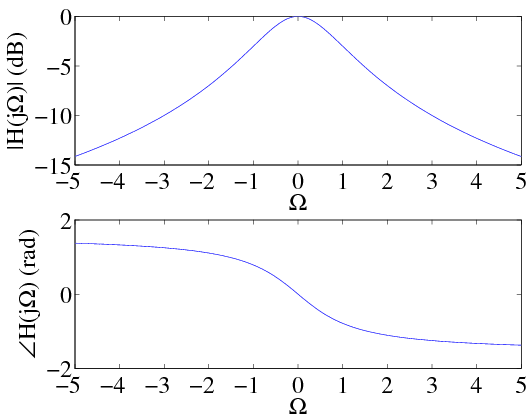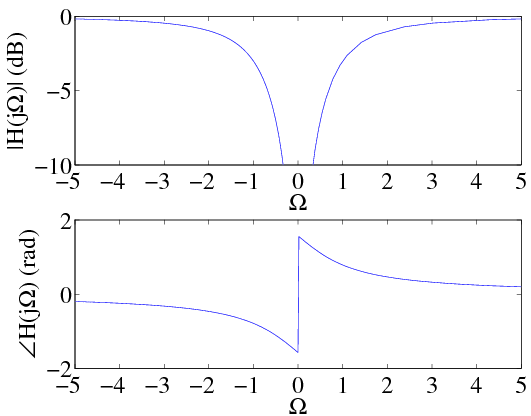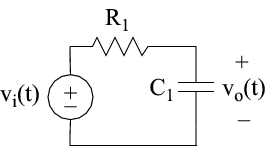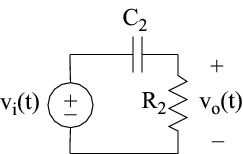# 3.13 First order filters

 Page 1 / 1

A first-order lowpass filter has the frequency response

${H}_{LP}\left(j\Omega \right)=\frac{1}{1+j\frac{\Omega }{{\Omega }_{c}}}$

The frequency at which the frequency response magnitude has dropped to $1/\sqrt{2}$ is called the corner frequency This term most likely originates from it's role in Bode plots, a shortcut method for sketching the graph of a frequency response. . The frequency response magnitude and phase are plotted in [link] . It is common to express the frequency response magnitude in units of decibels (dB) using the formula

$20lo{g}_{10}\left|H,\left(,j,\Omega ,\right)\right|$

At the corner frequency for a first order lowpass filter, the frequency response magnitude is $1/\sqrt{2}$ or roughly -3 dB. From [link] , it can easily be seen that the impulse response for the first-order lowpass filter is given by

${h}_{LP}\left(t\right)={\Omega }_{c}{e}^{-{\Omega }_{c}t}u\left(t\right)$

A first-order highpass filter is given by

${H}_{HP}\left(j\Omega \right)=\frac{j\frac{\Omega }{{\Omega }_{c}}}{1+j\frac{\Omega }{{\Omega }_{c}}}$

Notice that

${H}_{HP}\left(j\Omega \right)=1-\frac{1}{1+j\frac{\Omega }{{\Omega }_{c}}}$

This makes sense since a highpass filter can be constructed by taking the filter input $x\left(t\right)$ and subtracting from it a lowpass filtered version of $x\left(t\right)$ . The impulse response of the first-order highpass filter therefore becomes:

${h}_{HP}\left(t\right)=\delta \left(t\right)-{\Omega }_{c}{e}^{-{\Omega }_{c}t}u\left(t\right)$Frequency response magnitude and phase for a first-order lowpass filter ( Ω c = 1 rad/sec).Frequency response magnitude and phase for a first-order highpass filter ( Ω c = 1 rad/sec).

First order filters can be easily implemented using linear circuit elements like resistors, capacitors, and inductors. [link] shows a first order filter based on a resistor and a capacitor. Since the impedance for a resistor and capacitor are $R$ and $1/j\Omega C$ , respectively, voltage division leads to a frequency response of

${H}_{LP}\left(j\Omega \right)=\frac{1}{1+j\Omega {R}_{1}{C}_{1}}$

Therefore the corner frequency for this filter is ${\Omega }_{c}=\frac{1}{{R}_{1}{C}_{1}}$ . Similarly, a first-order highpass filter can be implemented using a resistor and capacitor as shown in [link] . This filter has a frequency response of

${H}_{HP}\left(j\Omega \right)=\frac{j\Omega {R}_{2}{C}_{2}}{1+j\Omega {R}_{2}{C}_{2}}$

The corner frequency for the highpass filter is seen to be ${\Omega }_{c}=\frac{1}{{R}_{2}{C}_{2}}$ .Circuit implementation of a first-order lowpass filter having Ω c = 1 / R 1 C 1 .Circuit implementation of a first-order highpass filter having Ω c = 1 / R 2 C 2 .

Now one might be tempted to apply the results of [link] to build a bandpass filter by cascading the lowhpass and highpass circuits in Figures [link] and [link] , respectively. Theory would predict that the equivalent frequency response of this circuit is given by

${H}_{eq}\left(j\Omega \right)={H}_{LP}\left(j\Omega \right){H}_{HP}\left(j\Omega \right)$

Unfortunately, this is not possible since the circuit elements in the lowpass and highpass filters interact with one another and therefore affect the overall behavior of the circuit. This interaction between the two circuits is called loading will be studied in greater detail in the exercises. To get theoretical behavior, it is necessary to use a voltage follower circuit, between the lowpass filter from the highpass circuits. The voltage follower circuit is usually an active circuit (requires external power supply) that has very high input impedance and very low output impedance. This eliminates any loading effects which would normally occur between the lowpass and highpass filter circuits.

are nano particles real
yeah
Joseph
Hello, if I study Physics teacher in bachelor, can I study Nanotechnology in master?
no can't
Lohitha
where we get a research paper on Nano chemistry....?
nanopartical of organic/inorganic / physical chemistry , pdf / thesis / review
Ali
what are the products of Nano chemistry?
There are lots of products of nano chemistry... Like nano coatings.....carbon fiber.. And lots of others..
learn
Even nanotechnology is pretty much all about chemistry... Its the chemistry on quantum or atomic level
learn
da
no nanotechnology is also a part of physics and maths it requires angle formulas and some pressure regarding concepts
Bhagvanji
hey
Giriraj
Preparation and Applications of Nanomaterial for Drug Delivery
revolt
da
Application of nanotechnology in medicine
has a lot of application modern world
Kamaluddeen
yes
narayan
what is variations in raman spectra for nanomaterials
ya I also want to know the raman spectra
Bhagvanji
I only see partial conversation and what's the question here!
what about nanotechnology for water purification
please someone correct me if I'm wrong but I think one can use nanoparticles, specially silver nanoparticles for water treatment.
Damian
yes that's correct
Professor
I think
Professor
Nasa has use it in the 60's, copper as water purification in the moon travel.
Alexandre
nanocopper obvius
Alexandre
what is the stm
is there industrial application of fullrenes. What is the method to prepare fullrene on large scale.?
Rafiq
industrial application...? mmm I think on the medical side as drug carrier, but you should go deeper on your research, I may be wrong
Damian
How we are making nano material?
what is a peer
What is meant by 'nano scale'?
What is STMs full form?
LITNING
scanning tunneling microscope
Sahil
how nano science is used for hydrophobicity
Santosh
Do u think that Graphene and Fullrene fiber can be used to make Air Plane body structure the lightest and strongest. Rafiq
Rafiq
what is differents between GO and RGO?
Mahi
what is simplest way to understand the applications of nano robots used to detect the cancer affected cell of human body.? How this robot is carried to required site of body cell.? what will be the carrier material and how can be detected that correct delivery of drug is done Rafiq
Rafiq
if virus is killing to make ARTIFICIAL DNA OF GRAPHENE FOR KILLED THE VIRUS .THIS IS OUR ASSUMPTION
Anam
analytical skills graphene is prepared to kill any type viruses .
Anam
Any one who tell me about Preparation and application of Nanomaterial for drug Delivery
Hafiz
what is Nano technology ?
write examples of Nano molecule?
Bob
The nanotechnology is as new science, to scale nanometric
brayan
nanotechnology is the study, desing, synthesis, manipulation and application of materials and functional systems through control of matter at nanoscale
Damian
Is there any normative that regulates the use of silver nanoparticles?
what king of growth are you checking .?
Renato
Got questions? Join the online conversation and get instant answers!

#### Get Jobilize Job Search Mobile App in your pocket Now!By OpenStaxBy Brooke DelaneyBy Michael PittBy OpenStaxBy Madison ChristianBy OpenStaxBy OpenStaxBy OpenStaxBy Robert MurphyBy OpenStax